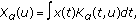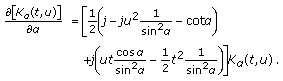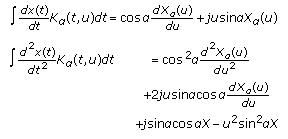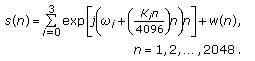# A New LFM-Signal Detector Based on Fractional Fourier Transform

## Abstract

A new LFM-signal detector formulated by the integration of the 4th-power modulus of the fractional Fourier transform is proposed. It has similar performance to the modulus square detector of Radon-ambiguity transform because of the equivalence relationship between them. But the new detector has much lower computational complexity in the case that the number of the searching angles is far less than the length of the signal. Moreover, it is proved that the new detector can be generalized to the integration of the n th-power () modulus of the fractional Fourier transform via mathematical derivation. Computer simulation results have confirmed the effectiveness of the proposed detector in LFM-signal detection.

## 1. Introduction

The detection of the Linear Frequency Modulation (LFM) signal is very important in many information systems, such as communications, radar, and sonar, for its wide use in these systems. In recent years, several time-frequency-based methods for LFM-signal detection have been proposed. Several intelligible detection algorithms using the short-time Fourier transform (STFT) or wavelet transform are mentioned in [1, 2]. However, the poor resolution according to the narrow or time-variant window used in the analysis limits their applications in practice. The discrete chirp-Fourier transform is employed in  to estimate the chirp rate of LFM-signal. But its rigorous constraint, that signal length must be prime number and the chirp rate must be integer, limits its application. Since the LFM-signal distributes as a straight line in the Wigner-Ville distribution (WVD) plane, the Radon-Wigner transform (RWT) algorithm computes integrals along the lines with different angles and positional offsets in the time-frequency plane to detect the LFM-signal [4, 5], and a two-dimensional search is necessary to track the straight lines in the time-frequency plane. The lines, whose integral values exceed a certain threshold, correspond to the LFM-signal. Similar to WVD, the ambiguity function (AF) of the LFM-signal distributes as a straight line passing through the origin in the AF plane. Therefore, Radon-ambiguity transform is also used to detect the LFM-signal, especially in the case that the chirp rate is the only parameter of interest, and two kinds of detectors (the envelope detector and the modulus square detector) have been studied in . For Radon-Wigner transform or Radon-ambiguity transform, both the calculation of the full time-frequency plane (or AF plane) and the transformation from the Cartesian coordinate to the polar coordinate are indispensable, whose high computational complexity severely hinders the usefulness of the two methods in the LFM-signal detection, especially in the case of long signal detection. Recently, as a new time-frequency analysis tool, the fractional Fourier transform (FRFT) attracts more and more attention in signal processing field [7, 8]. Several methods based on FRFT have been proposed to detect LFM-signal and estimate its parameters . An effective method for parameter estimation and recovery of time-varying signals including LFM-signal by using FRFT is proposed in . According to the equivalence relationship between RWT and the fractional power spectrum [13, 14], a LFM-signal detection and parameters estimation method based on FRFT has been presented [10, 11], which achieves a near-RWT performance at much lower computational complexity.

Similar to WVD, ambiguity function also has a close relation to FRFT [12, 15, 16]. Then an LFM-signal detector using fractional autocorrelation, which corresponds to the envelope detector of Radon-ambiguity transform, is proposed . In this paper, we propose a new LFM-signal detector formulated by the integration of the 4th-power modulus of the fractional Fourier transform. The new detector is equivalent to the modulus square detector of Radon-ambiguity transform and performs similarly as well in LFM-signal detection. But the new detector has much lower computational complexity in the case of long signal detection, or in the case that the possible distribution area of the signal in the ambiguity plane is limited to a small-angle sector. Mathematical derivation proves that the new detector can be generalized to the integration of the n th-power () modulus of the fractional Fourier transform.

The structure of this paper is as follows. In Section 2, a simple review of the definition of FRFT and its relation to AF are given. The modulus square detector of Radon-ambiguity transform is briefly introduced in Section 3. The new detector based on FRFT is proposed and its computational complexity compared with Radon-ambiguity transform is discussed in Section 4. Section 5 gives the mathematical derivation of the generalization of the new detector. Computer simulations are given in Section 6 to show the effectiveness of the proposed detector. Finally, conclusions are made in Section 7.

## 2. Definition of FRFT and Its Relation to AF

FRFT is a generalization of the standard Fourier transform. The FRFT of signal x(t) with angle α is defined by [7, 8](1)

whereis the transformation kernel,(2)

There are some interesting properties of FRFT corresponding to the transformation kernel [7, 8]. Here, we just list a few of them, which will be successfully employed in our mathematical derivation in the following sections:(3)(4)

The standard Fourier transform is an operator that transforms a time domain signal into a frequency domain representation. In time-frequency plane, Fourier transform can be interpreted as acounterclockwise rotation operator from the time axis to the frequency axis. FRFT, as the generalization of the standard Fourier transform, can be considered as a counterclockwise rotation of the signal coordinate around the origin on the u axis with an arbitrary angle, and transforms a signal to an intermediate domain between time and frequency. Therefore, FRFT can be classified into the time-frequency analysis tools, and it is strongly related to other important time-frequency transforms, such as WVD and AF.

For its perfect energy localization properties, AF is a very important and commonly used time-frequency analysis tool. The AF of signal x(t) is defined as(5)

By combing AF and Radon transform, a new LFM-signal detection method called Radon-ambiguity transform has been established . It is more efficient than RWT in the case that the chirp rate is the only parameter of interest.

Similar to WVD, AF also has simple but important relation to FRFT, and the transformation between them is easily expressed by a simple formula [15, 16].(6)

Equation (6) reveals the relationship between AF and FRFT as follows: the slice of AF at anglein the ambiguity plane is the Fourier transform of the fractional power spectrum of angle. Based on this relationship, an LFM-signal detection and chirp rate estimation method using FRFT, which has similar performance but lower computational complexity compared with Radon-ambiguity transform, is proposed in this paper.

## 3. The Modulus Square Detector of Radon-Ambiguity Transform

For an LFM-signal,(7)

Its AF can be obtained by substituting (7) into the definition formula of AF expressed by (5),(8)

It is obvious that the AF of LFM-signal has its energy concentrated along a ridge that passes through the origin in the ambiguity plane. The Radon transform is a well-performed tool to actually compute the integral values along the lines. Thus, by applying the Radon transform to AF, the LFM-signal detection is implemented by computing the line integral of AF along all the lines passing through the origin, which is well-known as the Radon-ambiguity transform method. Two different detectors of LFM-signal are proposed in . One of them is the modulus square detector(9)

The detector's good performance is discussed in detail and its usefulness in LFM-signal detection has been well demonstrated by some numerical examples in .

## 4. The New Detector Based on FRFT

AF calculated by (5) is a representation in the Cartesian coordinate. Contrarily, the "Radon transform" operation should be implemented in the polar coordinate. Thus, the transformation from the Cartesian coordinate to the polar coordinate is required in the implementation of Radon-ambiguity transform. To avoid this stubborn transformation, AF is calculated directly in the polar coordinate via FRFT according to the relationship between FRFT and AF expressed by (6). A new detector for LFM-signal, which is equivalent to the modulus square detector of Radon-ambiguity transform, can be derived. Substituting (6) into (9), the modulus square detector can be rewritten as(10)

Exchanging the order of integration, we get(11)

Then a new detector for LFM-signal is obtained, which is the integration of the 4th-power modulus of the fractional Fourier transform,(12)

The equivalence between the new detector and the modulus square detector of Radon-ambiguity transform can be easily demonstrated from the derivation process. For LFM-signal, the new detectorwill reach its maximum when the angleis equal to –arc cot(K), where K is the chirp rate of the LFM-signal. According to the equivalence relationship between the new detector and the modulus square detector of Radon-ambiguity transform, they have the nearly same performance except the computational complexity in theory.

We assume that the signal length is N and the number of the angles to search is M. The computation of Radon-ambiguity transform consists of the calculation of AF, the transformation from the Cartesian coordinate to the polar coordinate, and the Radon transformation . The calculation of AF in the full of the ambiguity plane consists of N-multiple fast Fourier transform, and has a computational complexity of. The computational complexity of the coordinate transformation depends on the details of implementation. The computational complexity of the Radon transformation is O(MN). Thus the total computational complexity of Radon-ambiguity transform is more than. The computation of the new method proposed in this paper consists of M-multiple fractional Fourier transform and the integration operation. Recently, several algorithms for the calculation of FRFT with different accuracies and computational complexities have been proposed . In this paper, we adopt the decomposition algorithm proposed in , which can be implemented by FFT and has a computational complexity of. Taking the computational complexity of the integration operation into consideration, the total computational complexity of the new method is just only. It can be seen that the computational complexity of the new method is much lower than that of Radon-ambiguity transform when the number of the searching angles M is far less than the length of the signal), which is most likely to occur in the case of long signal detection, or in the case that the distribution area of the signal in the ambiguity plane is limited to a small-angle sector, or in the case that the possible chirp rate of the signal is limited to a certain range. Figure 1 shows the comparison of the variation of computational complexity for the two methods with the increase of signal length N and number of searching anglesM. Obviously, as N and M () increases, the computational advantage of the new method becomes more and more significant.

## 5. The Generalization of the New Detector

An interesting phenomena, that the effective detector is not only limited to the integration of the 4th-power modulus of the fractional Fourier transform, but can also be generalized to n th-power modulus for, is found in our simulation. In this section, the mathematical derivation is implemented to prove it.

The generalized detector is written as follows:(13)

The effectiveness of the detector defined by (13) will be demonstrated, if the condition given as follows is satisfied:(14)

where K is the chirp rate of the LFM-signal.

Thus the differential of the detector with respect to the variable α is necessary to work out,(15)

Since the kernel function K α (t,u) is the only item that contains the variable α in the definition formula of FRFT expressed by (1), the differential of the FRFT X α (u) with respect to the variable α can be written as(16)

From (2), the differential of the kernel function K α (t,u) with respect to the variable α is(17)

Therefore(18)

Substituting the property of FRFT expressed by (3) into (18), we can obtain(19)

Considering the real part of (19) and omitting the imaginary part, the following equation can be obtained:(20)

For LFM-signal, the first- and second-order differential in time domain is expressed as follows, respectively,(21)(22)

Implementing FRFT operation to both sides of (21) and (22), we get(23)(24)

Substituting the property of FRFT expressed by (3) into the right side of (23) and (24), the FRFT of the second-order differential of the LFM-signal can be obtained as:(25)

Meanwhile, another form of the FRFT of the differential of the LFM-signal can be derived using the temporal differential property of FRFT expressed by (4), respectively,(26)

Combining (23), (25), and (26) the second order differential of FRFT of LFM-signal in fractional Fourier domain is obtained and expressed by(27)

Substituting (27) into (20), we can obtain(28)

By making the following change:, (28) can be rewritten as the following form:(29)

Substituting (29) into (15), an ordinary differential equation of the detector function can be obtained.(30)

Combining the initial condition(31)

The solution of the ordinary differential equation is obtained.(32)

It can be seen that, for LFM-signal, the generalized detectorwill reach its maximum corresponding to the chirp rate. Therefore, the generalized detector can be regarded as an effective detector for LFM-signal. However, the 4th-power modulus form detector is most frequently used in practice for its lower computational complexity compared with other generalized forms.

## 6. Simulation Results

In this section, some simulation examples, which are exactly the same as the ones used in [6, 12], are presented to verify the effectiveness of the new method for LFM-signal detection. In example_I, a discrete single-component LFM-signal is used as the reference signal:(33)

Here the chirp rate is.is a complex additive noise, generated via two independent, zero-mean, Gaussian random processes of equal variance. Figure 2 shows the outputs of the detector calculated via (12) with signal-to-noise ratio (SNR) equaling to, −6 dB, −9 dB, respectively, for different chirp rate values.

In example_II, a discrete multicomponent LFM-signal is used as the reference signal:(34)

The initial frequency and chirp rate parameter values are as follows:,,,,,,,. Figure 3 displays the detection output calculated via (12) with SNR equaling to, −6 dB, −12 dB, respectively, for different chirp rate values,. It can be seen from Figures 2 and 3 that the results agree with those in . The peaks of the detection output occur at the chirp rate K corresponding to the LFM components, and there is an explicit one-to-one correspondence between the peaks and the chirp rates. All of the LFM components with correct chirp rate are accurately detected, and the two closely spaced chirp rates atandare also well resolved. The two simulations above have verified the effectiveness of the proposed detector formulated by the integration of the 4th-power modulus of the fractional Fourier transform.

Figures 4 and 5 show the detection outputs of the 3rd and 5th-power modulus detectors with SNR equaling to −6 dB, respectively. It is obvious that the peak value over noise floor in Figure 5 is higher than that in Figure 4. Then the conclusion can be drawn that the peaks are easier to pick in the noise floor as the order number of power modulus increases. However, the higher order power modulus also inhibits the smaller signals when the detected signal consists of multicomponent LFM-signal with different amplitudes. It means that we should carefully choose the form of generalized detectors according to specific conditions in the practical applications of the new method.

## 7. Conclusions

This paper presents a new detector of LFM-signal, which is the integration of the 4th-power modulus of its fractional Fourier transform. The new detector has equivalence relation to the modulus square detector of Radon-ambiguity transform, and performs similarly to Radon-ambiguity transform in LFM-signal detection under much lower computational complexity. Moreover, it has been proved mathematically that the new detector can be generalized to the integration of the n th-power modulus of the fractional Fourier transform for. Computer simulations have verified the effectiveness of the new detectors.

## References

1. Altes RA: Detection, estimation, and classification with spectrograms. Journal of the Acoustical Society of America 1980, 67(4):1232-1246. 10.1121/1.384165

2. Sanghadasa M, Erbach PS, Sung CC, Gregory DA, Friday WA: Wavelet transform applied to synthetic aperture radar-optical implementation and adaptive techniques. Optical Engineering 1994, 33(7):2282-2289. 10.1117/12.172241

3. Xia X-G: Discrete chirp-Fourier transform and its application to chirp rate estimation. IEEE Transactions on Signal Processing 2000, 48(11):3122-3133. 10.1109/78.875469

4. Rao P, Taylor FJ: Estimation of instantaneous frequency using the discrete Wigner distribution. Electronics Letters 1990, 26(4):246-248. 10.1049/el:19900165

5. Wood JC, Barry DT: Radon transformation of time-frequency distributions for analysis of multicomponent signals. IEEE Transactions on Signal Processing 1994, 42(11):3166-3177. 10.1109/78.330375

6. Wang M, Chan AK, Chui CK: Linear frequency-modulated signal detection using radon-ambiguity transform. IEEE Transactions on Signal Processing 1998, 46(3):571-586. 10.1109/78.661326

7. Ozaktas HM, Zalevski Z, Kutay MA: The Fractional Fourier Transform with Applications in Optics and Signal Processing. Wiley, Chichester, UK; 2001.

8. Tao R, Qin L, Wang Y: Theory and Applications of the Fractional Fourier Transform. Tsinghua University Press, Beijing, China; 2004.

9. Serbes A, Durak L: Optimum signal and image recovery by the method of alternating projections in fractional Fourier domains. Communications in Nonlinear Science and Numerical Simulation 2010, 15(3):675-689. 10.1016/j.cnsns.2009.05.013

10. Qi L, Tao R, Zhou S, Wang Y: Detection and parameter estimation of multicomponent LFM signal based on the fractional Fourier transform. Science in China. Series F 2004, 47(2):184-198. 10.1360/02yf0456

11. Dorsch RG, Lohmann AW, Bitran Y, Mendlovic D, Ozaktas HM: Chirp filtering in the fractional Fourier domain. Applied Optics 1994, 33(32):7599-7602. 10.1364/AO.33.007599

12. Akay O, Boudreaux-Bartels GF: Fractional convolution and correlation via operator methods and an application to detection of linear FM signals. IEEE Transactions on Signal Processing 2001, 49(5):979-993. 10.1109/78.917802

13. Almeida LB: Fractional fourier transform and time-frequency representations. IEEE Transactions on Signal Processing 1994, 42(11):3084-3091. 10.1109/78.330368

14. Lohmann AW, Soffer BH: Relationships between the Radon-Wigner and fractional Fourier transforms. Journal of the Optical Society of America A 1994, 11(6):1798-1801. 10.1364/JOSAA.11.001798

15. Ozdemir AK, Arikan O: Fast computation of the ambiguity function and the Wigner distribution on arbitrary line segments. IEEE Transactions on Signal Processing 2001, 49(2):381-393. 10.1109/78.902121

16. Alieva T, Bastiaans MJ, Stanković L: Signal reconstruction from two close fractional fourier power spectra. IEEE Transactions on Signal Processing 2003, 51(1):112-123. 10.1109/TSP.2002.806593

17. Ozaktas HM, Ankan O, Kutay MA, Bozdagi G: Digital computation of the fractional fourier transform. IEEE Transactions on Signal Processing 1996, 44(9):2141-2150. 10.1109/78.536672

18. Vargas-Rubio JG, Santhanam B: On the multiangle centered discrete fractional Fourier transform. IEEE Signal Processing Letters 2005, 12(4):273-276.

19. Pei S-C, Yeh M-H, Tseng C-C: Discrete fractional Fourier transform based on orthogonal projections. IEEE Transactions on Signal Processing 1999, 47(5):1335-1348. 10.1109/78.757221

## Acknowledgments

This work is supported by the China Postdoctoral Science Foundation under Grant 20090460726. The authors would like to thank the anonymous reviewers who give some valuable comments for improving the quality of this paper.

## Author information

Authors

### Corresponding author

Correspondence to Weidong Chen.

## Rights and permissions

Reprints and Permissions

Yin, Z., Chen, W. A New LFM-Signal Detector Based on Fractional Fourier Transform. EURASIP J. Adv. Signal Process. 2010, 876282 (2010). https://doi.org/10.1155/2010/876282

• Revised:

• Accepted:

• Published:

• DOI: https://doi.org/10.1155/2010/876282

### Keywords